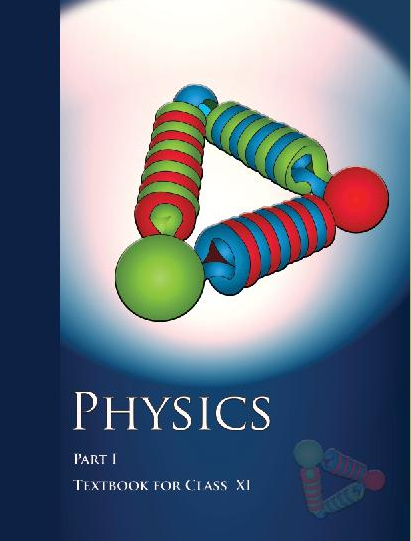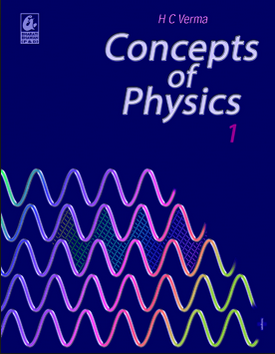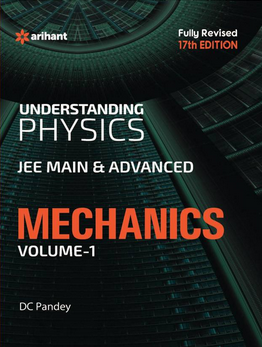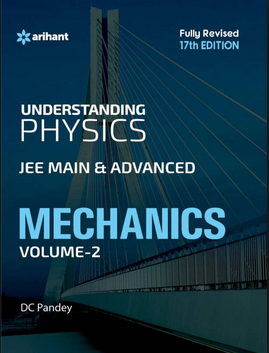# Rotational Motion (Weightage 3%)   Share

### Topics from Rotational Motion

• Rigid body rotation (1 concepts)
• Basic concepts of rotation (2 concepts)
• Moment of Inertia introduction (3 concepts)
• Values of moments of inertia for simple geometrical objects (9 concepts)
• Parallel and Perpendicular axes theorems and its applications (2 concepts)
• Radius of Gyration (1 concepts)
• Equationof rotational motion (14 concepts)
• Angular momentum and its applications (5 concepts)
• Centre of massof two-particle system (2 concepts)
• Centre of mass of rigid body (16 concepts)
• Rigid body and its centre of Mass (13 concepts)
• Basic concepts of rotational motion (3 concepts)
• Conservation of angular momentum and its applications (3 concepts)
• Moment of Inertia (12 concepts)
• Combined rotation and translation motion (4 concepts)

### Important Books for Rotational Motion

••••Exams
Articles
Questions